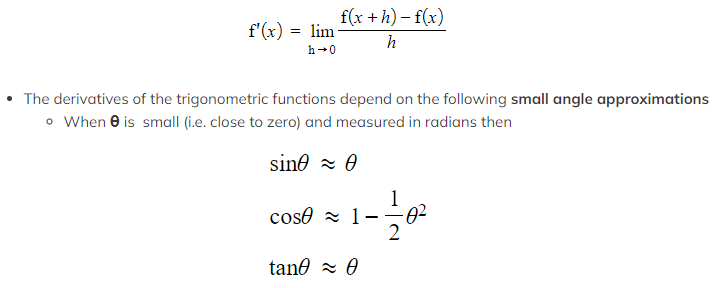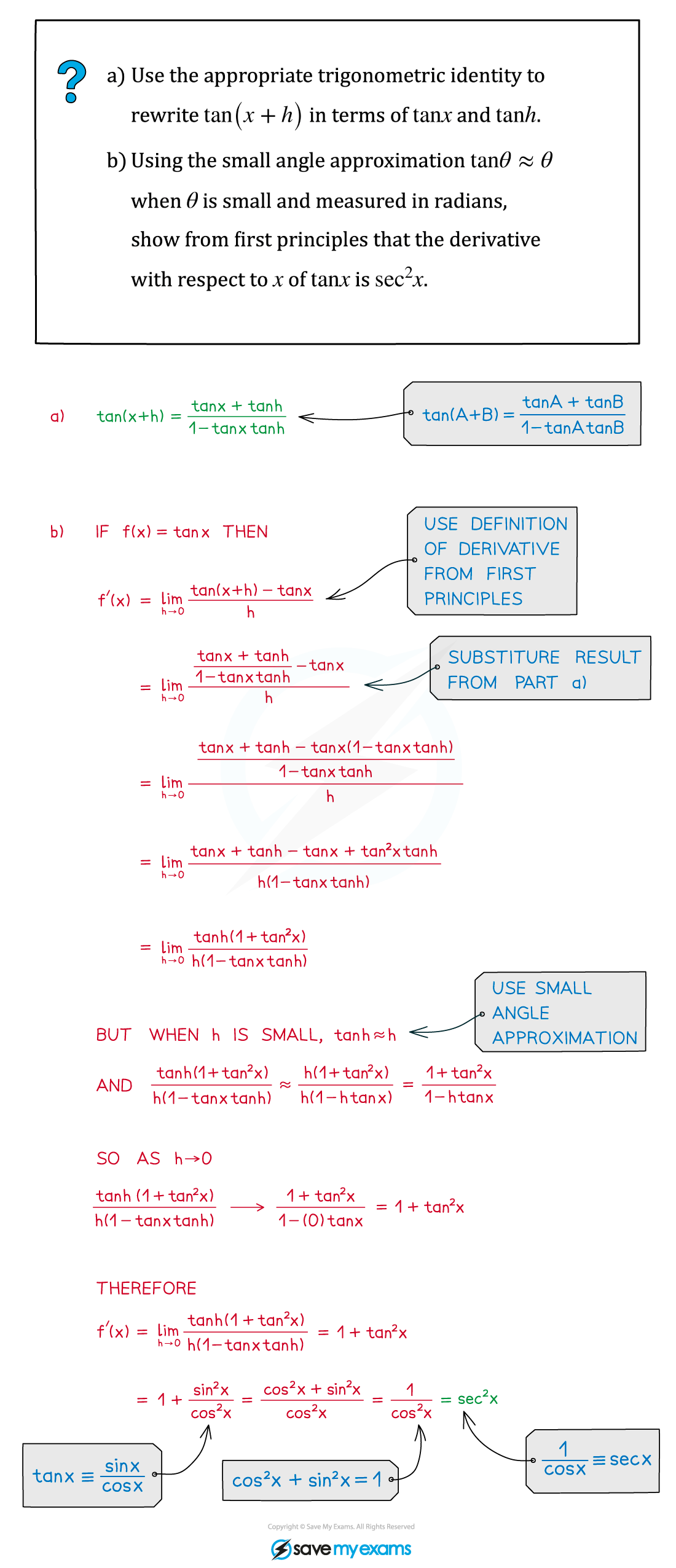# AQA A Level Maths: Pure复习笔记7.3.1 First Principles Differentiation - Trigonometry

### First Principles Differentiation - Trigonometry

#### How do I derive the derivatives of trigonometric functions from first principles?

• Recall that for a function f(x) the definition of the derivative from first principles (see First Principles Differentiation) is:The small angle approximations allow us to produce the following intermediate limit results:• And those intermediate results allow us to find the derivatives of sin and cos#### Derivatives of other trigonometric functions

• The derivative of tan is given by the following formula:• The easiest way to derive this is to use the quotient rule and the derivatives of sin and cos
• But it can also be derived from first principles using the small angle approximation for tan (see the Worked Example)
• The general formulae for the derivatives of the trigonometric functions are:• These formulae follow from combining the derivatives of the three basic functions with the chain rule, but they are worth knowing on their own

#### Exam Tip

• Remember that when doing calculus with trigonometric functions you have to measure angles in radians.
• The formula for the derivative of tan x is included in the exam formulae booklet.
• The derivatives of sin x and cos x are NOT included in the formula booklet – you have to know them.
• The small angle approximations for cos xsin x and tan x are included in the exam formulae booklet – you don't have to memorise them.
• Be sure to read first principle differentiation exam questions clearly – they will state any results you can treat as 'givens' in your answer.

#### Worked Example